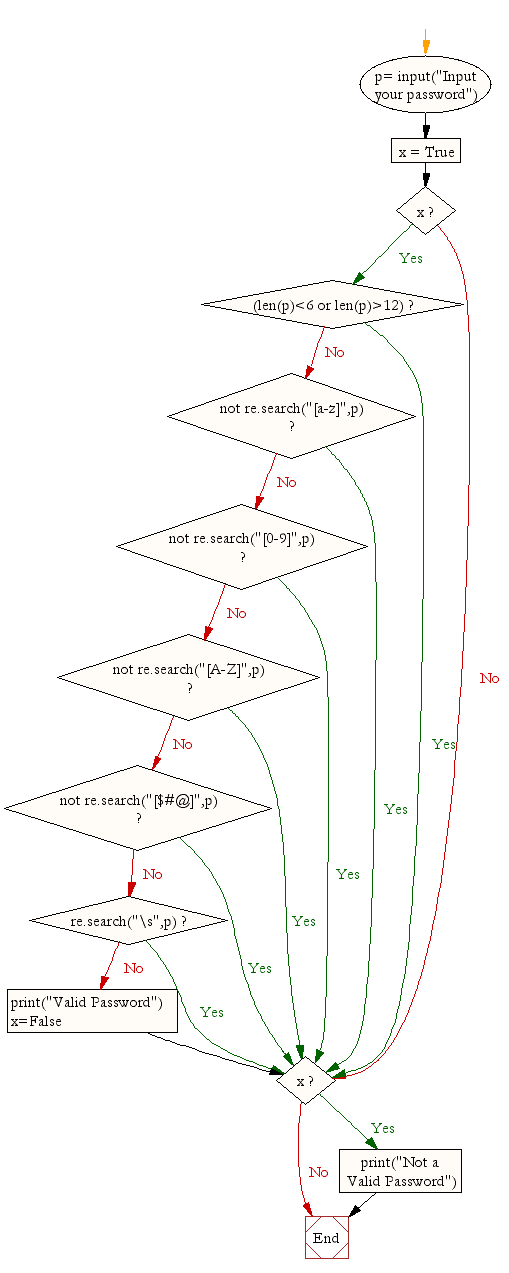﻿ Python Exercise: Check the validity of a password - w3resource# Python Exercise: Check the validity of a password

## Python Conditional: Exercise-15 with Solution

Write a Python program to check the validity of a password (input from users).

Validation :

• At least 1 letter between [a-z] and 1 letter between [A-Z].
• At least 1 number between [0-9].
• At least 1 character from [\$#@].
• Minimum length 6 characters.
• Maximum length 16 characters.

Sample Solution:-

Python Code:

``````import re
x = True
while x:
if (len(p)<6 or len(p)>12):
break
elif not re.search("[a-z]",p):
break
elif not re.search("[0-9]",p):
break
elif not re.search("[A-Z]",p):
break
elif not re.search("[\$#@]",p):
break
elif re.search("\s",p):
break
else:
x=False
break

if x:
```
```

Sample Output:

```Input your [email protected]
```

Flowchart:## Visualize Python code execution:

The following tool visualize what the computer is doing step-by-step as it executes the said program:

Python Code Editor:

Have another way to solve this solution? Contribute your code (and comments) through Disqus.

What is the difficulty level of this exercise?

Test your Python skills with w3resource's quiz

﻿

## Python: Tips of the Day

Returns a list with n elements removed from the beginning

Example:

```def tips_take(itr, n = 1):
return itr[:n]
print(tips_take([1, 2, 3], 5))
print(tips_take([1, 2, 3], 0))
```

Output:

```[1, 2, 3]
[]
```